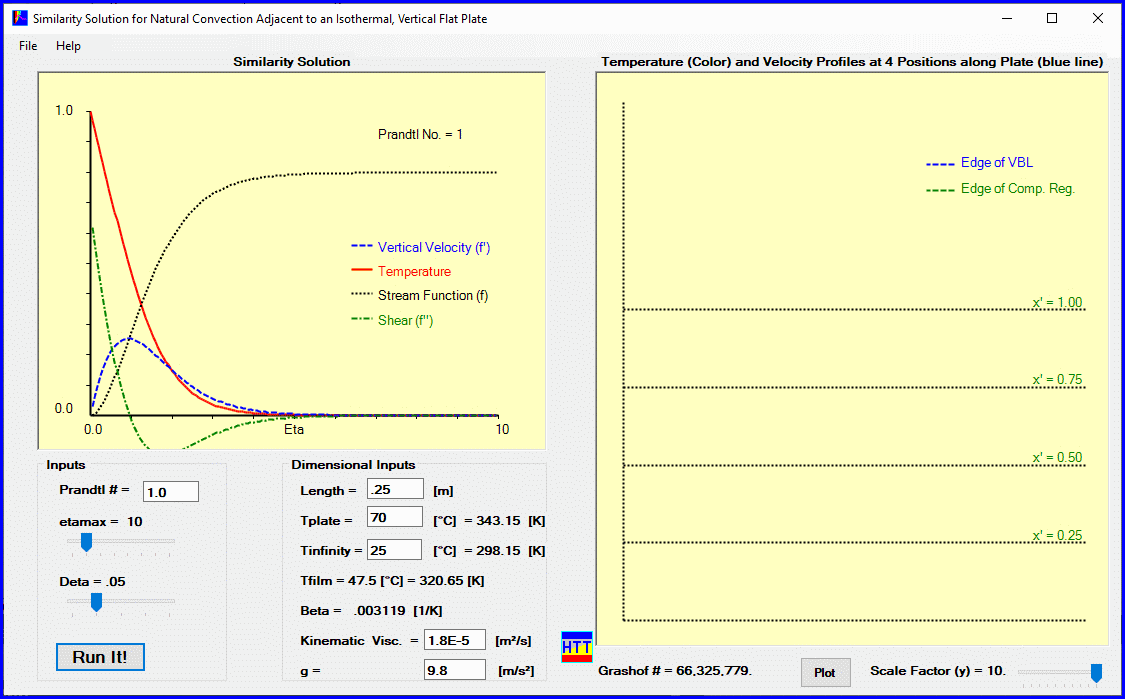# Natural Convection next to a Heated, Vertical Flat Plate

UPDATED: 08/04/2022

This module solves the governing equations for natural convection next to a heated vertical flat plate in their similarity form. The continuity, vertical momentum (simplified using the Boussinesq approximation) and thermal energy equations are converted to a pair of non-linear, coupled ordinary differential equations. With boundary conditions specified at both the surface of the plate and out at a distance from the plate, these equations are discretized and the resulting linear system is solved iteratively.

Module Description

The only inputs for the similarity solution are the Prandtl number of the fluid, the extent of the computing region (ηmax) and the spatial increment (Δη) to be used across the computing region.   Results in similarity form for vertical velocity and temperature are plotted in the left window.  To compute results in dimensional form the length and temperature of the plate and ambient temperature are input, as well as the kinematic viscosity of the fluid and the acceleration of gravity.   With that data, the vertical velocity profile is displayed in dimensional form at four stations along the plate in the right-hand window, where they are superimposed on the temperature field.  The effects of the Prandtl number of the fluid can be explored easily (and compared with results for forced convection over a flat plate). The value of existing analytical solutions covering extreme Prandtl numbers (Pr<<1 (liquid metals) and Pr>>1 (oils)) is evident from these calculations.   The traditional way of solving this problem is matched asymptotic expansions.Graphical user interface for natural convection adjacent to a vertical heated flat plate. User inputs are at the bottom left. The similarity solution is returned in the top left window and the dimensional solution for vertical velocity and temperature are shown in the right window.